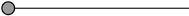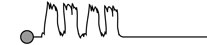> Français> English> Deutsch
 PRODUITS ET SERVICESRESSOURCESINFO TETRAEDREusername : password :

Proposal of April 30th, 2016. Thierry Schneider, Tetraedre Sarl

The maximum size of the LoRaWAN payload is 64 bytes. This limited size is the main reason to develop a flexible and compact data encoding.

Tetraedre is active in the Internet Of Things technology since many years and has developped efficient and versatile data encoding.

The goal is not to be the most size efficient but to find a good trade-off between size, versatility and portability.

The first byte of the payload is named header_main. This is the only fixed part of the payload. The description of this parameter is given below. The rest of the payload is a concatenation of data chunks. Chunks are either of type A, type B or type C. There might be any mix of chunks, in any order.

 LoRaWAN Payload First byte rest of payload header_main serie of chunks

The two must significant bits of header_main are reserved and must be set to 0. Allowed values for header_main are thus 0 to 63.

Chunk type distinction

Each chunk start with a header byte. This byte is used for data identification but also to know the size of the chunk.

Header value Type / Description
0x00 Reserved value. Means end of stream
0x01 - 0x5F Type A Chunk. Fixed size. 3 bytes
0x60 - 0x7F Type D Chunk. Fixed size. 2 bytes
0x80 - 0xBF Type B Chunk. Fixed size. 5 bytes
0xC0 - 0xFE Type C Chunk. Variable size
0xFF reserved value. Means end of stream

Type A Chunk

The type A chunk is 3 bytes long. The first byte is the chunk header. The header is followed by 2 bytes

byte8 is MSB

Type B Chunk

The type B chunk is 5 bytes long. The first byte is the chunk header. The header is followed by 4 bytes

 header databyte24 databyte16 databyte8 databyte0

byte24 is MSB

When data are transmitted in floating point the IEEE754 format must be used

Type C Chunk

The type C chunk is a variable size block. The first byte is the chunk header. The header is followed the size parameter which indicates the size of the data field

 header size data fieldlength is "size" bytes

Type D Chunk

The type D chunk is 2 bytes long. The first byte is the chunk header. The header is followed by 1 byte

Content definition

The content is defined with a combination of header_main and of header. The following table is a proposal of standard values.

0 1 0x01 A Temperature. signed integer 16bits. 1 LSB = 0.01°C.   0: 0°C; 1: +0.01°C; 0xFFFF : -0.01°C
0 2 0x02 A Relative Humidity. unsigned integer 16bits. 1 LSB = 0.01°RH
0 3 0x03 A Oxygen concentration. 1 LSB = 0.001%
0 4 0x04 A CO2 concentration. 1 LSB = 0.001%
0 5 0x05 A Second temperature. signed integer 16bits. 1 LSB = 0.01°C.   0: 0°C; 1: +0.01°C; 0xFFFF : -0.01°C
0 6 0x06 A Pressure. unsigned integer 16bits. 1 LSB = 0.5mbar
0 7 0x07 A analog channel #0. 1 LSB = 1 uA
0 8 0x08 A analog channel #1. 1 LSB = 1 uA
0 9 0x09 A analog channel #2. 1 LSB = 1 uA
0 10 0x0A A analog channel #3. 1 LSB = 1 uA
0 11 0x0B A digital inputs state
0 12 0x0C A relative pulse counter 0
0 13 0x0D A relative pulse counter 1
0 14 0x0E A relative pulse counter 2

0 16 0x10 A analog channel #0. 1 LSB = 1 mV
0 17 0x11 A analog channel #1. 1 LSB = 1 mV
0 18 0x12 A analog channel #2. 1 LSB = 1 mV
0 19 0x13 A analog channel #3. 1 LSB = 1 mV

0 or 1 96
0x60
D Battery voltage
if (value>=81)
Volt = 4.2 + (value-80) * 0.1
else
Volt = 1.8 + value * 0.03
1 97
0x61
D M-BUS meter status byte

0 or 1 128  0x80 B timestamp of the measurement. unsigned integer 32 bits. UNIX timestamp
1 129 0x81 B Main energy index. IEEE754 floating point. Unit : kWh for electricity meters (register 1.8.0)
1 130 0x82 B Serial number. unsigned integer 32bits. Serial number of the meter
1 131 0x83 B First tariff energy index. IEEE754 floating point. Unit : kWh for electricity meters (register 1.8.1)
1 132 0x84 B Second tariff energy index. IEEE754 floating point. Unit : kWh for electricity meters (register 1.8.2)
1 133 0x85 B Main energy index. IEEE754 floating point. Unit : m3 for water meter
1 134 0x86 B Main energy index. IEEE754 floating point. Unit : m3 for uncorrected gaz meters
1 135 0x87 B Flow temperature. IEEE754 floating point. Unit : °C
1 136
0x88
B absolute pulse counter 0. unsigned integer 32bits
1 137
0x89
B absolute pulse counter 1. unsigned integer 32bits
1 138
0x8A
Power register. IEEE754 floating point. Unit W.
1 139
0x8B
B Main energy index. IEEE754 floating point. Unit : kWh for heat meter
1 164 B Pressure. IEEE754 floating point. Unit : mbar

1 192 0xC0 C ZMD410 profil. First is timestamp (32bits unix) followed by either 1, 2 or 3 float16 values. The values definition and number are dependant of the ZMD410 configuration. Their encoding is float16 (see below). The first value represents to the first field of the profil, the second value (if any) represents the second field,...
1 200 0xC8 C M-BUS data chunk.
1

201

0xC9

C

For water meter

status information + Last read index + array of index differences (delta)

 4 bytes 1 byte 4 bytes n x 2 byte meter SNR 32-bits integer MSB first status information last read index 32-bit float32 MSB array of indexes each index is 16-bit

Status information:

length: 8-bits

format:

 bit7 bit6 bit5 bit4 bit3 bit2 bit1 bit0 reserved configuration: 000 = acq interval of 3600 seconds (1 hour) 001 = acq interval of 900 seconds (15 minutes) 010 = acq interval of 38400 seconds (1 day) meter battery error: 0 = no battery error 1 = battery error meter other type of error: 0 = no other type of error 1 = one or more other type of error

Last index is 32-bit IEEE754 floating point MBS first value followed by n 16-bit float16 "delta" (as described in the chapter "Type definition", section "float16 type" after the present table). Note that if the "delta" value is 0xFFFF, it means that its value is invalid.

The first value is the most recent value. The second value is the second most recent value ... and so on.

Note that if the most recent value (the index) is invalid (because we couldn't read the meter properly for example), it will be replaced by only 2 bytes 0xFFFF value. The valid index might (or not) be inserted later in the data or replaced by 0xFFFF. Once a valid index has been provided, only delta values are provided in the data. This means that if the float32 (IEEE754) value starts with 0xFFFF, it is an invalid delta (and the index might appear somewhere else )

See "Profile decoding" in the "Example" section after the present table.

1

202

0xCA

C

Format similar to header 201, except that this header is used gas meter

1 224 0xE0 C Index of the FastForward EnergyCam module.
1 229 0xE5 C  SNR of the FastForward EnergyCam module.

If you intend to use this specification or if you have comments, please inform me.

Type definition

float16 type

The goal of the float16 type is to encode small and large values using only 16-bit. Of course this is not as efficient as IEEE754 but it already gives interesting possibilites. Only positive values are accepted. Values in range 0 to 181930 are converted.

The two most significant bits define a decimal range (0.001, 0.2, 1, 5) (exponent), while the 14 less significant bits defines the value (mantisse). Use the following code to decode the values:

left = (value >> 14) & 0x03;
value0 = value & 0x3FFF;
result = (float32) value0;
switch(left)
{
case 0:
result = result * 0.001;
break;
case 1:
result = result * 0.02;
result = result + 16.38;
break;
case 3:
result = result * 5;
result = result + 16725;
break;
case 2:
default:
result = result + 344;
break;
}

VBAT type

The goal of the VBAT type is to encode the battery voltage with a single byte and sufficient accuracy.

The decoding is very simple: V is the byte value.

if (v<128)
Voltage = 1 + ((v & 0x7F) * 0.025)
else
Voltage = 4.175 + ((v & 0x7F) * 0.15)

if (v==0)
Voltage = 0; // undefined

Examples

Profile decoding

Example index without delta:

01 80 5b 6d 63 b0 82 00 12 d6 87 ca 0b 00 43 2a 00 00 ff ff ff ff ff ff

80 timestamp

5b 6d 63 b0 UNIX timestamp = 2018-08-10 12:06:40 GMT+2

82 serial number

00 12 d6 87 hexa =1234567 decimal

ca header = uncorrected gas meter

0b size = 11. Unit: bytes

00 conf = 0000 0000 = acq interval of 3600 seconds (1 hour) & no battery error & 0 = no other type of error

43 2a 00 00 index IEE754 Floating point MSB first = 170 decimal. Unit: m3

ff ff delta 1

ff ff delta 2

ff ff delta 3

Example with delta:

01 80 5b 6d 68 68 82 00 12 d6 87 ca 0b 00 43 34 00 00 02 58 01 2c 00 64

80 timestamp

5b 6d 68 68 2018-08-10 12:26:48 GMT+2

82

00 12 d6 87 hexa =1234567 decimal

0b size = 11

00 conf = 0000 0000 = acq interval of 3600 seconds (1 hour) & no battery error & 0 = no other type of error

43 34 00 00 = index IEE754 Floating point MSB first = 180 decimal. Unit: m3

02 58 delta 1 hex value = 0000 0010 0101 1000 binary

left = (value >> 14) & 0x03;

this means: (0000 0010 0101 1000 >> 14) == 00

then apply the "and" mask

00

&11

---------------

result: 00

value0 = value & 0x3FFF;

0000 0010 0101 1000

& 0011 1111 1111 1111

result: 0000 0010 0101 1000

result = 0b0000001001011000 = 0x258 =0d600

result = (float32) value0;

result = (float32) 0d600;

result = 600.00

switch (left)

case 0

result = result / 100.0;

=> result = 600.00/100.00 == 6.00

Delta 1 = 6.00 m3

As last index = 180 m3

The previous index can be calculated as last index – delta 1 = 180 – 6 = 174 m3and it was the index reas 1 hour ago as conf == 00